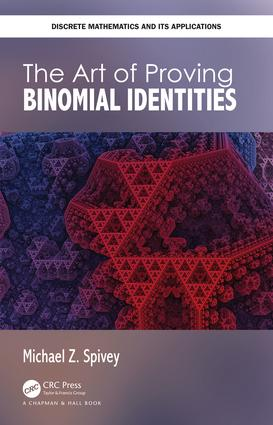The Art of Proving Binomial Identities

1st Edition

Chapman and Hall/CRC

368 pages | 29 B/W Illus.

Purchasing Options:\$ = USD
Hardback: 9780815379423
pub: 2019-05-14
SAVE ~\$17.99
\$89.95
\$71.96
x
eBook (VitalSource) : 9781351215824
pub: 2019-05-10
from \$44.98

FREE Standard Shipping!

Description

The Art of Proving Binomial Identities accomplishes two goals: (1) It provides a unified treatment of the binomial coefficients, and (2) Brings together much of the undergraduate mathematics curriculum via one theme (the binomial coefficients). The binomial coefficients arise in a variety of areas of mathematics: combinatorics, of course, but also basic algebra (binomial theorem), infinite series (Newton’s binomial series), differentiation (Leibniz’s generalized product rule), special functions (the beta and gamma functions), probability, statistics, number theory, finite difference calculus, algorithm analysis, and even statistical mechanics.

The book is very suitable for advanced undergraduates or beginning graduate students and includes various exercises asking them to prove identities. Students will find that the text and notes at the end of the chapters encourages them to look at binomial coefficients from different angles. With this learning experience, students will be able to understand binomial coefficients in a new way.

Features:

• Provides a unified treatment of many of the techniques for proving binomial coefficient identities.
• Ties together several of the courses in the undergraduate mathematics curriculum via a single theme.
• A textbook for a capstone or senior seminar course in mathematics.
• Contains several results by the author on proof techniques for binomial coefficients that are not well-known.
• Ideal for self-study, it contains a large number of exercises at the end of each chapter, with hints or solutions for every exercise at the end of the book.

Introducing the Binomial Coefficients. Basic Techniques. Combinatorics. Calculus. Probability. Generating Functions. Recurrence Relations and Finite Differences. Special Numbers. Miscellaneous Techniques. Hints and Solutions to Exercises.

Michael Z. Spivey is Professor of Mathematics at the University of Puget Sound, where he currently serves as chair of the Department of Mathematics and Computer Science. He earned his PhD in operations research from Princeton University. He has authored more than 25 mathematics papers, most of which are on optimization, combinatorics, or the binomial coeffcients.